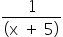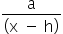Mathematics
Easy

Question

# What are the equations of the asymptotes of the function? f(x) =– 3

## x = 5 and y = 3x = -5 and y = -3 x = 3 and y = -5x = -3 and y = 5Hint:

## The correct answer is: x = -5 and y = -3

### We have to find the equation of the asymptotes.For the function, f(x) =+ kHorizontal asymptote: y = k Vertical asymptote: x = h In this case, asymptotes are x = -5 and y = -3.So, the asymptotes to the graph f(x) = 1/(x+5) - 3 are x = -5 and y = -3.Hence, the correct option is A.

The vertical asymptote is y= k and the horizontal asymptote is x=h, where (h,k) is a moving point on the graph.

### Related Questions to study#### With Turito Foundation.#### Get an Expert Advice From Turito.